Students often turn to OP Malhotra Class 9 Maths Solutions Chapter 8 Triangles Chapter Test to clarify doubts and improve problem-solving skills.

## S Chand Class 9 ICSE Maths Solutions Chapter 8 Triangles Chapter Test

Question 1.
(i) Which of the following is not a criterion for congruency of triangles?
(a) SAS
(b) ASA
(c) SSA
(d) SSS
(ii) Which congruence statement correctly indicates that the two given triangles are congruent?
(a) ∆ABC ≅ ∆EFD
(b) ∆ABC ≅ ∆DEF
(c) ∆ABC ≅ ∆FDE
(d) ∆ABC ≅ ∆FED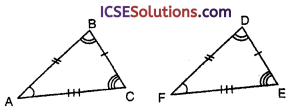Solution:
(i) Among the given criterion
SSA is not a criterion
Others are criterion

(ii) Fom the given figure,
AC = FE
AB = FD
BC = DE
∴ ∆ABC ≅ ∆FDE

Question 2.
(i) Show that ∆RST ≅ ∆TUR when x = 18.
(ii) Show that ∆ABC ≅ ∆DBC when x = 4.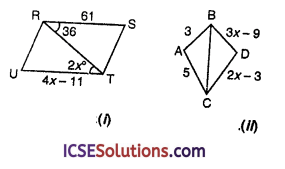Solution:
(i) When x = 18, then
4x – 11 = 4 x 18 – 11 = 72 – 11
= 61 ⇒ RS = UT = 61
and 2x = 2 x 18 = 36°
⇒ ∠SRT = ∠RTU = 36°
RT = RT (common)
Hence ∆RST ≅ ∆TUR (SAS criterion)

(ii) In the figure,
ABDC is a kite in which AB = DB
⇒ 3x – 9 = 3 ⇒ 3x = 3 + 9 = 12
⇒ x = $$\frac { 12 }{ 3 }$$ = 4
AC = DC
⇒ 5 = 2 x 4 – 3 ⇒ 5 = 8 – 3 = 5
BC = BC (common)
∴ ∆ABC ≅ ∆DBC (SSS criterion)Question 3.
D is a point on the side BC of a ∆ABC such that AD bisects ∠BAC. Then,
(a) BD = CD
(b) BA>BD
(c) BD > BA
(d) CD > CA
Solution:
In ∆ABC,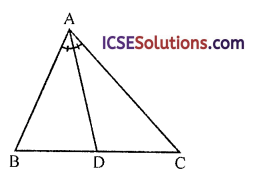D is apoint on BC and AD bisects ∠BAC
∴ In ∆ABD
BA > BD

Question 4.
Two sides of a triangle are of lengths 6 cm and 2.6 cm. The lengths of the third side of the triangle cannot be
(a) 4.7 cm
(b) 5 cm
(c) 4.9 cm
(d) 3.2 cm
Solution:
Two sides of ∆ABC,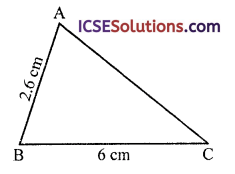Let BC = 6 cm and AB = 2.6 cm
Now BC – AB = 6 – 2.6 = 3.4 cm
∴ Third side must be greater than 3.4 cm
∵ Sum of any two sides is greater than third side
∴ 3.2 cm can be the third side.

Question 5.
In ∆ABC, ∠B = 30°, ∠C = 80° and ∠A = 70°, then
(a) AB > BC < AC
(b) AB < BC > AC
(c) AB > BC > AC
(d) AB < BC < AC
Solution:
In ∆ABC, ∠B = 30°, ∠C = 80°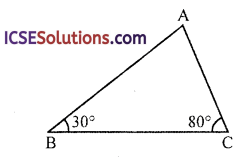Then ∠A = 180° – (30° + 80°) = 180° – 110° = 70°
Then AB > BC > ACQuestion 6.
Two sides of a triangle are of lengths 4 cm, and 10 cm. If the lengths of the third side is ‘a’ cm, then
(a) a > 5
(b) 6 ≤ a ≤ 12
(c) a < 6
(d) 6 < a < 14
Solution:
In ∆ABC, two sides are 4 cm and 10 cm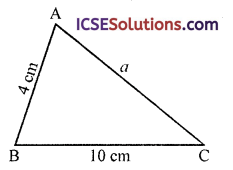Let AB = 4 cm and BC = 10 cm ‘a’ is third side
∵ Sum of any two sides > third side
10 < 4 + a ⇒ 10 – 4 < a ⇒ 6 < a
and a < 4 + 10 ⇒ a < 14
∴ 6 < a < 14